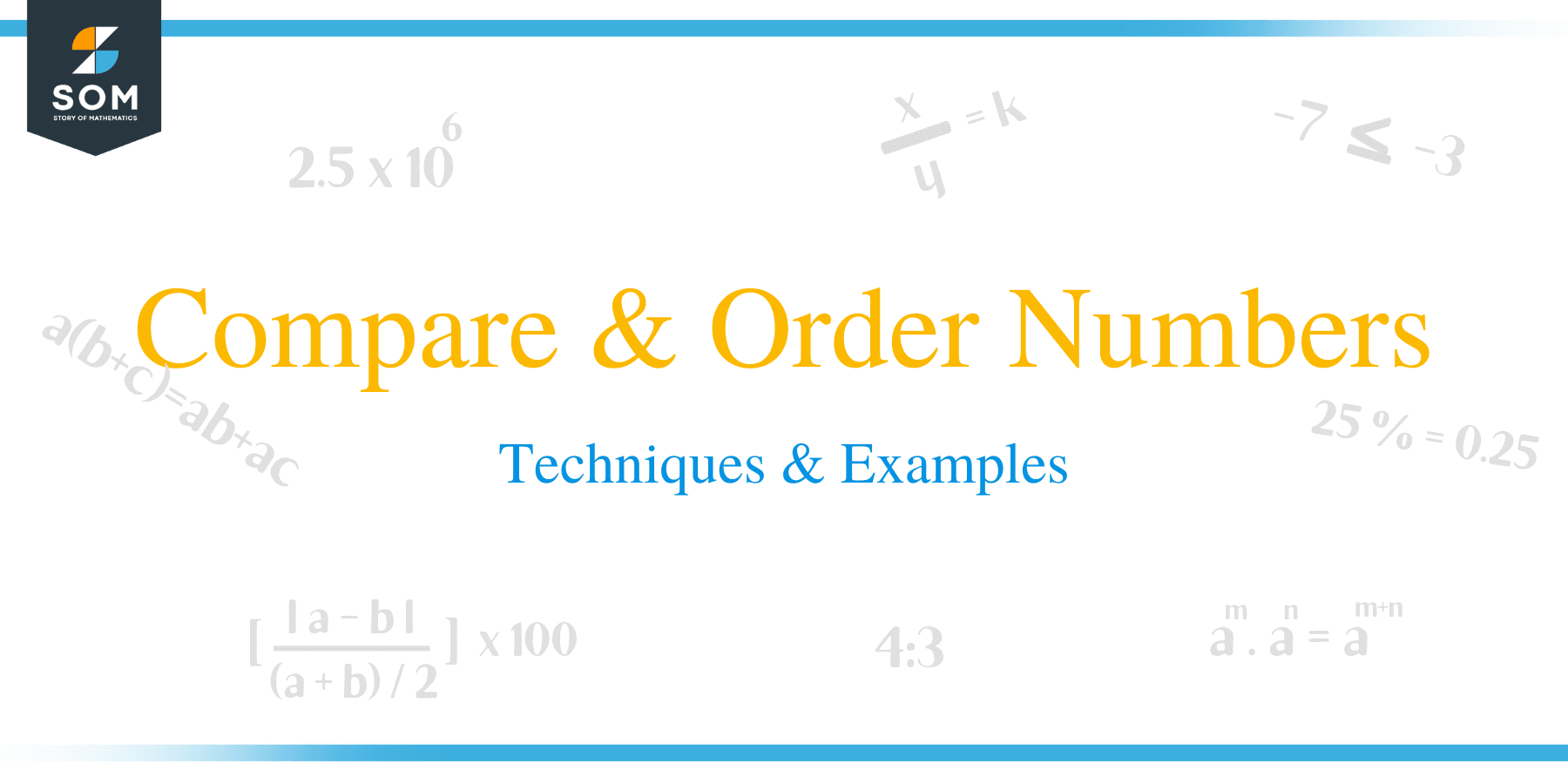# Compare & Order Numbers – Techniques & Examples

##How to Compare Numbers?

The most important thing to know when comparing and ordering numbers is the value of the most significant digit in each of the numbers.

The most significant digit will let you the size of each number. The most significant digit in a number is the first non-zero integer of a number. The larger the digit, the bigger the number, but unless the is negative.

For example, in the number 57,467, the most significant digit is ‘5’, which has the place value of 50000.

In the number 43.786, the most significant integer is 4, which has a place value of 40. The most significant digit in 0.0754 is 7, with a place value of 0.07.It is easier to compare positive whole numbers just by looking at the digits of the number.

When comparing numbers, we often use these symbols like > < and = . Comparing In general, if a and b are two numbers: a > indicates that number a is greater than number b;

a < b shows that a is less than b, and lastly, a = b shows that number a is equivalent to number b.

Examples 1

a. 245 > 200 means that 245 is greater than 200

b. 23 < 86 means that 23 is less than 86

c. 45 = 20 +25 means that 45 is equivalent to 20 + 7

### Comparing Numbers Amounting to 10

It is very simple to compare numbers amounting to 10. You look for phrases such as the largest, biggest, least, less than, and greater.

### Comparing numbers Amounting to 100

The value of the tens digit checked when comparing numbers up to 100. The larger the number in the tens digit, the bigger the number.

If both numbers have the same digit in the tens value, then the one digit is checked to identify which number is more.

Example 2

a. 98 > 56 means that 98 is greater than 56

b. 67 < 88 means that 67 is less than 88 c. 95 > 91 means that 95 is greater than 91

### Comparing Numbers up to 1000

When comparing a three-digit number or number up to 999, the value of the hundreds digit checked. In this case, the hundreds digit is the most significant digit for a three-digit number. The larger the digit in the hundreds digit, the bigger the number.

If the numbers have the same digit in the hundreds value, then the next numbers, such as tens and ones, are checked to identify which number is more.

Example 4

a. 825 > 824 means 825 is greater than 824
The ones digit in the first number has a higher value than the ones digit in the second number; therefore, it is bigger.

b. 486 < 491 means 486 is less than 491 since the hundreds digits are equal in the two numbers, so we look at the tens digit. The second number has the higher value of the tens digit. c. 203 > 28 means that, 203 is greater than 28

You can notice that the second number has no hundreds digit, and therefore, it is smaller.

### How to Compare Negative Numbers?

Comparison of negative numbers is a bit complicated, but operation been simplified b the following rules:

• For negative numbers the more the number becomes, the lesser its value.
• Shifting to the right of a number line increases the value of negative numbers.
• Shifting to left along the number line, decreases the value of negative numbers.
• Zero and any positive number is always greater than a negative number.

Example 4

0 > – 5 means that, zero is greater than -5

– 9 < -3 means that, -9 is less than -3 -54 > -86 means that, – 54 is greater than -86

-39 < 1 means that, -39 is less than 1

## How to Order Numbers?

When ordering numbers, we first start by comparing two numbers at a time. You can do this by either arranging the numbers in ascending or descending order.Example 5

Arrange the following numbers in ascending order:

4679; 4542; 4797; 4545

Solution

Each number has 4 in thousands digit, then move to hundreds digit;

4797 is the biggest and 4679 the smallest number.

4542 and 4545 have the same value in the tens digit.

Comparing the ones place, 4545 > 4542

There the ascending order is 4542 < 4545 < 4679 < 4797

Example 6

Arrange the numbers in descending order:

42593; 70537; 38524; 67198

Solution

Compare digits according to place value.

And so, the descending order is: 70537 > 67198 > 42593 > 38524

### Practice Questions

1. Is the inequality, $386 > 300$, true or false?

2. Is the inequality, $43 < 56$, true or false?

3. Is the inequality, $40 + 3 > 43$, true or false?

4. Is the inequality, $128 > 136$, true or false?

5. Is the inequality, $87 < 98$, true or false?

6. Is the inequality, $734 > 733$, true or false?

7. Is the inequality, $578 > 581$, true or false?

8. Is the inequality, $0 > – 8$, true or false?

9. Is the inequality, $-12 < -8$, true or false?

10. Which of the following shows the correct order of the set, $\{5328, 5349, 5304, 5324, 5298\}$, in ascending order?

11. Which of the following shows the correct order of the set, $\{4567, 6574, 4426, 5897, 5908, 5780\}$, in descending order?